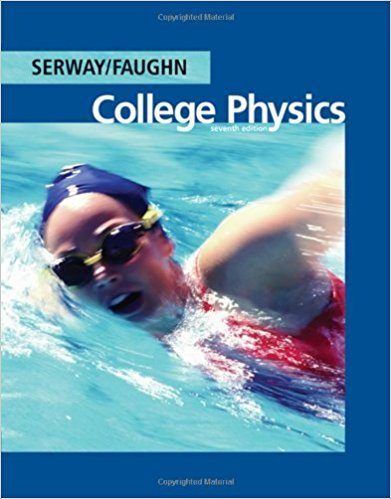×
Get Full Access to College Physics - 7 Edition - Chapter 18 - Problem 18.48
Get Full Access to College Physics - 7 Edition - Chapter 18 - Problem 18.48

×

# Three 60.0-W, 120-V lightbulbs are connected across aISBN: 9780495113690 154

## Solution for problem 18.48 Chapter 18

College Physics | 7th Edition

• Textbook Solutions
• 2901 Step-by-step solutions solved by professors and subject experts
• Get 24/7 help from StudySoup virtual teaching assistantsCollege Physics | 7th Edition

4 5 1 417 Reviews
10
3
Problem 18.48

Three 60.0-W, 120-V lightbulbs are connected across a 120-V power source, as shown in Figure P18.48. Find (a) the total power delivered to the three bulbs and (b) the potential difference across each. Assume that the resistance of each bulb is constant (even though, in reality, the resistance increases markedly with current).

Step-by-Step Solution:
Step 1 of 3

Chapter 22: Magnetism Bar magnets have poles on opposite sides called north poles and south poles. They act as normal magnets (like attracts like, opposites repel) Magnetic field lines exit from the north pole and enter from the south. F= |q|vBsin(thet) Magnitude of the Magnetic Force: o SI unit is newton (N) o q is the charge o v is the velocity o B is the magnetic field Right hand rule o Source: Google images/ boundless.com Since the magnetic force is always perpendicular to the direction of motion, the path of a particle is always circular. o The radius is travels: r=(mv)/( |q|B) Magnetic force on a current carrying wire: F=ILBsin (

Step 2 of 3

Step 3 of 3

##### ISBN: 9780495113690

Unlock Textbook Solution